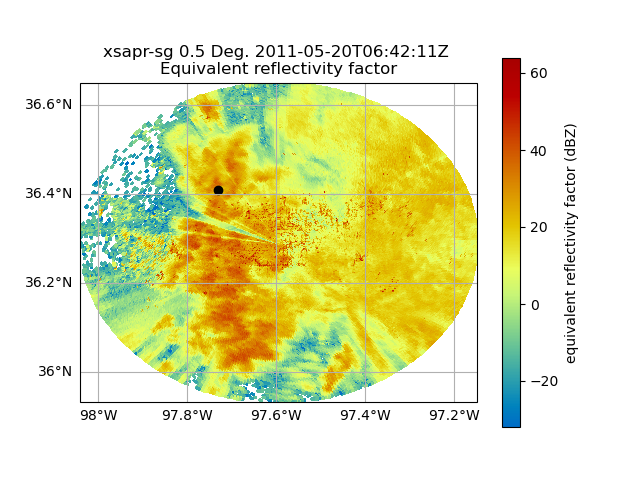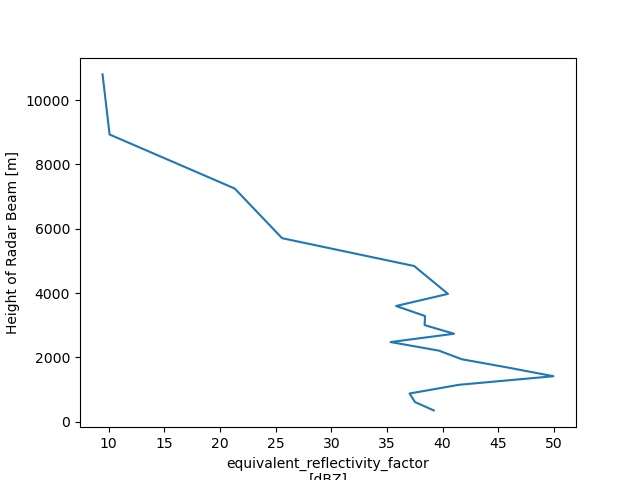# Extract a radar column above a point#

Given a radar and a point, extract the column of radar data values above a point

```# Author: Maxwell Grover (mgrover@anl.gov)

import cartopy.crs as ccrs
import matplotlib.pyplot as plt
import numpy as np

import pyart
from pyart.testing import get_test_data

# Read in some test data
filename = get_test_data("swx_20120520_0641.nc")
```

Plot the first sweep and our desired point

Let’s visualize our radar data from a single sweep, and plot the location of our desired point on a map. This will provide some context as to where we are extracting our column of values.

```site_lon = -97.73  # longitude in degrees
site_lat = 36.41  # latitdue in degrees

ax = plt.subplot(111, projection=ccrs.PlateCarree())

# Visualize the reflectivity field, using the lowest sweep with
# latitude and longitude lines
display.plot_ppi_map(
"reflectivity_horizontal",
0,
ax=ax,
vmin=-32,
vmax=64.0,
lon_lines=np.arange(-98, -97, 0.2),
lat_lines=np.arange(36, 37, 0.2),
)

# Plot our site location on top of the radar image
ax.scatter(site_lon, site_lat, color="black")
``````<matplotlib.collections.PathCollection object at 0x7f7fa0b56b10>
```

Now that we have our point defined, and our radar object, we can use the following utility function in Py-ART to subset a column

```ds = pyart.util.columnsect.get_field_location(radar, site_lat, site_lon)
```

This function returns an xarray dataset, with all of our data fields!

```print(ds)
```
```<xarray.Dataset>
Dimensions:                            (height: 22)
Coordinates:
* height                             (height) float64 350.6 ... 1.818e+04
Data variables: (12/15)
corrected_reflectivity_horizontal  (height) float64 39.2 37.52 ... nan nan
reflectivity_horizontal            (height) float64 32.88 31.46 ... nan nan
recalculated_diff_phase            (height) float64 1.555 1.32 ... -0.0 -0.0
specific_attenuation               (height) float64 0.2778 0.1806 ... 0.0
unf_dp_phase_shift                 (height) float32 58.05 56.55 ... 36.27
mean_doppler_velocity              (height) float32 -9.133 -9.953 ... 5.688
...                                 ...
dp_phase_shift                     (height) float64 145.5 144.0 ... nan nan
diff_reflectivity                  (height) float32 0.0 0.0 0.0 ... 0.0 0.0
proc_dp_phase_shift                (height) float32 51.8 50.25 ... 36.27
copol_coeff                        (height) float64 0.95 0.92 ... nan nan
base_time                          datetime64[ns] 2011-05-20T06:42:11
time_offset                        (height) datetime64[ns] 2011-05-20T06:...
Attributes:
azimuth:                233.545 degrees
latitude_of_location:   36.41 degrees
longitude_of_location:  -97.73 degrees
```

Visualize the Reflectivity Values in the Column

Let’s visualize the reflectivity values in the column above our point, which is stored in our new dataset

```ds.corrected_reflectivity_horizontal.plot(y="height")
``````[<matplotlib.lines.Line2D object at 0x7f7fa0c01e90>]
```

Total running time of the script: (0 minutes 3.020 seconds)

Gallery generated by Sphinx-Gallery